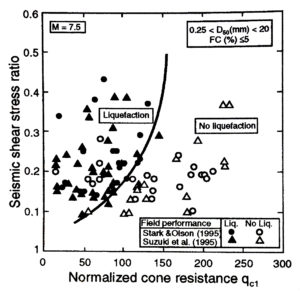In our last blog, we discussed using the CPT to estimate the shear strength of soil, which helps gauge how soil will behave during changes in stress. One important application of this capability is the estimation of soil liquefaction potential, meaning the potential of soil to dramatically lose strength when subjected to changes in stress.

Liquefaction is of particular concern in sandy, saturated soils. Shaking due to an earthquake or other sudden force causes the grains of loosely packed, sandy soils to settle into a denser configuration. If the soil is saturated and the loading is rapid, pore water does not have time to move out of the way of settling soil: pore water pressure rises, effectively pushing the soil grains apart and allowing them to move more freely relative to each other. At this point, the soil can shift and flow like a liquid—hence the name liquefaction.

This dramatic reduction of soil stiffness and strength causes soil to shift under pre-existing forces—say, the pressure of a building’s foundation or the pull of gravity on a slope. The increased pore pressure also increases the force of the soil on in-ground structures such as retaining walls, dams, and bridge abutments.

How can the potential for these effects be evaluated using the CPT? The subject is complex, as the wealth of research on the subject over several decades shows! Many approaches for determining cyclic liquefaction potential rely on the cyclic stress ratio (CSR), which requires a seismic analysis of the site. It expresses the ratio of the average cyclic shear stress in an earthquake of a given magnitude and the effective vertical overburden stress at the test site.

### CSR = 0.65(MWF)(amax/g)(σvo/σ′vo)rd

Where:

MWF = Magnitude Weighting Factor = (Magnitude)2.56/173

amax = maximum ground surface acceleration

g = acceleration of gravity, 9.81m/s2

σvo = total vertical overburden stress

σ′vo = effective vertical overburden stress

rd = stress reduction factor, normally given rd = 1 – 0.015z, where z = soil depth in meters

Hang in there, we’re not done! In clean sand, the next step is to calculate the clean sand-corrected tip resistance, qc1:

### qc1 = (qc/pa)(pa/ σ′vo)0.5

Where:qc = tip resistance
pa = atmospheric pressure
σ′vo = effective vertical overburden stress

With these calculations in hand, we turn to historical data. We plot our values with CSR on the y-axis and qc1 on the x axis, along with historical data from sites where liquefaction effects were or were not observed following earthquakes with similar strength and soil conditions. The curves separating instances where liquefaction did and didn’t occur are known as CRR (cyclic resistance ratio) curves. In the example data shown above, a data point that falls on the CRR curve represents soil conditions with a 20% or smaller chance of liquefaction. Note that this approach assumes clean sand (i.e. a low fines content or low percentage of fine-grained particles), level ground, and low overburden stress: if conditions are otherwise, additional corrections are needed.
Though the calculations seem complicated, CPT testing is a comparatively straightforward method for estimating liquefaction potential. It benefits from a large volume of historical data, good repeatability, and great ease of data collection, and it allows soil conditions to be evaluated at continuous depths. Unlike laboratory soil tests, CPT testing results in minimal soil disturbance, so results are a good approximation of how the soil will behave in real-world conditions.